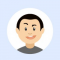## Write a Golang program to search an element in a sorted arrayPublished on 04-Feb-2021 11:12:12
Approach to solve this problemStep 1: Iterate the array from the 0th index to n-1, where n is the size of the given array.Step 2: Declare low=0th index and high=n-1. Start a for loop till low is lesser than high.Step 3: Find mid=(low+high)/2, if the element at the middle is ... Read More

## Write a Golang program to sort an array using Bubble SortPublished on 04-Feb-2021 11:10:05
Definition: Bubble Sort is the simplest sorting algorithm that works by repeatedly swapping the adjacent elements if they are in the wrong order.ExamplesInput arr = [7, 5, 1, 6, 3]1st iteration => swap(7, 5) => swap(7, 1) => swap(7, 6) => swap(7, 3) => [5, 1, 6, 3, 7]2nd iteration ... Read More

## Write a Golang program to find prime numbers in a given rangePublished on 04-Feb-2021 11:09:30
ExamplesInput num1=3 and num2=8 => Prime numbers are: 3, 5, 7Input num1=8 and num2=23 => Prime numbers are: 11, 13, 17, 19, 23Approach to solve this problemStep 1: Define a function that accepts two numbers, num1 and num2, type is int.Step 2: Iterate between num1 and num2.Step 3: If the ... Read More

## Write a Golang program to find the frequency of an element in an arrayPublished on 04-Feb-2021 11:08:26
ExamplesIn the Input array, arr = [2, 4, 6, 7, 8, 1, 2]Frequency of 2 in given array is 2Frequency of 7 is 1Frequency of 3 is 0.Approach to solve this problemStep 1: Define a function that accepts an array and a numStep 2: Declare a variable count = 0.Step ... Read More

## Write a Golang program to convert a decimal number to its binary formPublished on 04-Feb-2021 11:06:55
ExamplesInput decimal_num = 13 => Output = 8+4+1 => 1101Input decimal_num = 11 => Output = 8+2+1 => 1011Approach to solve this problemStep 1: Define a function that accepts a decimal number, decimal_num, type is int.Step 2: Define an array to store the remainder while dividing decimal number by 2.Step ... Read More

## Write a Golang program to convert a binary number to its decimal formPublished on 04-Feb-2021 11:06:23
ExamplesInput binary_num = 1010111 => decimal number = 64+0+16+4+2+1 = 87Input binary_num = 10101 => decimal number = 21Approach to solve this problemStep 1: Define a function that accepts a binary number, binary_num, declare decimalNum = 0, index = 0Step 2: Start for loop until binary_num becomes 0.Step 3: Find ... Read More

## Write a Golang program to calculate the sum of elements in a given arrayPublished on 04-Feb-2021 11:05:48
ExamplesInput arr = [1, 3, 4, 5, 6] => 1+3+4+5+6 = 19Input arr = [5, 7, 8, 9, 1, 0, 6] => 5+7+8+9+1+0+6 = 36Approach to solve this problemStep 1: Define a function that accepts an array.Step 2: Declare a variable, res = 0.Step 3: Iterate the array and add ... Read More

## Write a Golang program to reverse a numberPublished on 04-Feb-2021 11:03:01
ExamplesInput num = 1432 => output = 2341Input num = 9878 => output = 8789Input num = 6785 => output = 5876Approach to solve this problemStep 1: Define a function that accepts a number (num); type is int.Step 2: Define res = 0 variable and start a loop until num ... Read More

## Write a Golang program to reverse an arrayPublished on 04-Feb-2021 11:01:58
ExamplesInput arr = [3, 5, 7, 9, 10, 4] => [4, 10, 9, 7, 5, 3]Input arr = [1, 2, 3, 4, 5] => [5, 4, 3, 2, 1]Approach to solve this problemStep 1: Define a function that accepts an array.Step 2: Start iterating the input array.Step 3: Swap first ... Read More

## Write a Golang program to reverse a stringPublished on 04-Feb-2021 11:01:13
ExamplesInput str = “himalaya” => Reverse String would be like => “ayalamih”Input str = “mountain” => Reverse String would be like => “niatnuom”Approach to solve this problemStep 1: Define a function that accepts a string, i.e., str.Step 2: Convert str to byte string.Step 3: Start iterating the byte string.Step 4: ... Read More